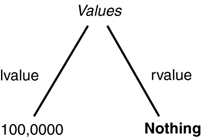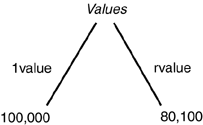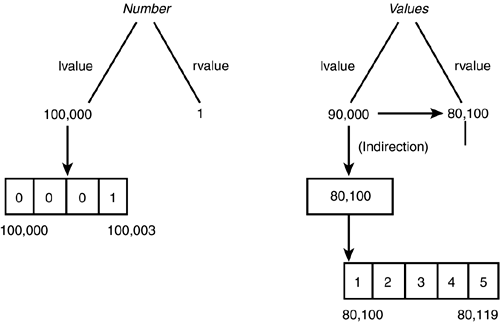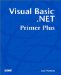# Arrays as Objects

Even though all arrays in Visual Basic .NET are actually objects, Visual Basic .NET provides a means for defining array objects that is different from those provided by other dialects of BASIC. We discussed arrays at considerable length in Chapter 7. However, that discussion was based on the standard way to define arrays common to all BASIC dialects. In this section, we explore the more explicit definition of array objects in Visual Basic .NET.

#### Reference Variables

The following two lines of code define an array object of type Integer :

` Dim Values As Integer()  Values = New Integer(5){} `

Notice that the syntax is a little different than we've seen before. The first statement creates a variable named Values . In our previous definition of an Integer array, Values was immediately followed by two parentheses. The parentheses enclosed a number that defined the highest index for the array.

This definition for Values is substantially different. The parentheses now follow the Integer keyword. Using this syntax causes Visual Basic .NET to place Values into the symbol table in a very different way than other BASICs would. Visual Basic .NET performs the usual checks of the symbol table (for example, whether Values already exists in the symbol table, and so on) and makes a request to the Windows memory manager for some storage. However, the storage request it makes is not for an integer array. Instead, Visual Basic .NET asks for enough storage to hold a reference variable. A reference variable is a special variable that is used to hold a memory address as its rvalue . Let's see what's really going on with these two statements.

As I mentioned, the first statement defines a variable named Values and identifies it as a reference variable. Visual Basic .NET asks Windows for enough memory to store one reference variable. Assuming that there is some free memory, Windows passes back an lvalue where Values can be stored. It records that lvalue in the symbol table and then makes a special note in the symbol table that this variable is a reference variable. It does this because Visual Basic .NET can do special things with reference variables that it can't do with other (non-reference type) variables.

After the first statement is completed, Values can be depicted as shown in Figure 14.8.

##### Figure 14.8. A representation of the Values reference variable.The rvalue is initialized by Visual Basic .NET to hold a unique value called Nothing . Actually, Nothing is a keyword in Visual Basic .NET and it used to indicate an empty rvalue for a reference variable. In Figure 14.8, we've assumed that Values exists in memory at location 100,000.

Programmer's TipReference variables are similar to pointer variables in other languages, like C++. In those languages, Nothing has the same interpretation as a Null pointer.

##### All Reference Variables Require the Same Storage

What isn't clear from Figure 14.8 is that all reference variables take the same amount of storage; 4 bytes for each reference variable. In other words, it wouldn't matter if Values were an Integer array or an array of Double s, the amount of storage for Values would always be the same. The reason is because a reference variable always holds the same thing: a memory address. That is, a valid reference variable will have an rvalue that is either a memory address or Nothing . That's it nothing else is allowed! Let's see why.

Let's look at the second statement:

` Values = New Integer(5){} `

When Visual Basic .NET sees this statement, it goes to the symbol table and looks for a reference variable named Values . If Visual Basic .NET doesn't find Values in the symbol table, you'll get an error message stating that Values is not declared. (If I were in charge of error messages at Microsoft, the message would say the variable is not defined. Given that the person with the most toys wins, I don't think I'm going to get this changed anytime soon.)

Assuming that Values is properly defined in the symbol table, Visual Basic .NET then sees the keywords New and Integer . The New keyword tells Visual Basic .NET we want to create a new object. The Integer keyword tells Visual Basic .NET what type of object to create. The 5 enclosed in parentheses tells Visual Basic .NET this is an array-of-integers object and that we want enough storage for six integer values. Don't forget, the parenthesized number is the highest index allowed, not the number of elements. In this example, it asks for elements 0 through 5 for a total of six elements. I'll explain what the curly braces (that is, the { and } ) mean in a moment.

After reading the entire statement, Visual Basic .NET knows the type of object you want (that is, an array of Integer s) and its size (that is, six integers). Visual Basic .NET then sends a message to Windows: "Hey, Windows! Visual Basic .NET here. I need enough storage for six integers. How's it looking?" Assuming that the memory is available, Windows sends a message back saying, "Hey, Visual Basic .NET! It's me, Windows. I found the storage you need. It's located at memory address 80,100." Visual Basic .NET then places that memory address into the symbol table as the rvalue for Values . Our memory image for Values now looks like that shown in Figure 14.9.

##### Figure 14.9. A representation of the Values reference variable after object creation.The important thing to notice in Figure 14.9 is that we've replaced the Nothing rvalue for Values with the memory address of where the object is stored in memory.

Note an important difference here between the old-style data definition and the style presented here. With the old style, the statement would have been

` Dim Number as Integer `

and Number would be an integer variable, not a reference variable. In this case, if Number had the same lvalue of 100,000, it would occupy memory locations 100,000 through 100,003. Any values held in Number would appear in the memory locations 100,000 through 100,003. (This isn't the case for the Values variable because it's an array.) Therefore, if Visual Basic .NET wants to access Number , it would simply go to the lvalue (that is, 100,000) and return the 4 bytes it finds there.

However, because Values is marked in the symbol table as a reference variable, Visual Basic .NET knows that the rvalue of Values isn't an integer value. Instead, the rvalue of Values is the memory address of where the integer data is found. With a reference variable, to find the value of the first element in the array, Visual Basic .NET reads the rvalue , jumps to that memory address, and fetches the first 4 bytes starting at memory address 80,100. The process of reading a memory address from an rvalue and then jumping to that memory address is called indirection. In other words, the memory address in the reference variable Values allows Visual Basic .NET to indirectly fetch the values of the integer array object after jumping to the proper memory address.

Programmer's TipAny of you who have taken the time to wade through the assembly language code will recognize that I've taken a few liberties in the precise mechanics of the way things work. However, I feel the simplification is warranted because it makes the explanation that follows a little clearer.

If all this seems like you spent the last 15 minutes talking with Yogi Berra, perhaps Figure 14.10 will help. First of all, let's assume that Number is defined as a simple integer number (not an array object). We'll further assume that it's stored in memory starting at address 100,000. For the Values reference variable, we'll assume that it's located at memory address 90,000, and Windows has located its object storage at memory address 80,100. We'll further assume that Number has the value of 1 and the first 5 elements of Values store the integer values 1 through 5.

##### Figure 14.10. The difference between defining an integer and defining an array object.Given those details, a memory image for both variables appears in Figure 14.10. Note that the figure on the left is for the standard Integer definition, whereas the image on the right is for the reference variable.

As you can see in Figure 14.10, the standard integer definition has an lvalue (100,000) that marks where the integer is stored in memory. Therefore, its rvalue actually is the value of the integer (1 in our example).

The reference to an array object is different. Because Values is a reference variable, its rvalue refers (or points) to the memory location where we find the first element of the array. Therefore, we fetch the rvalue (80,100) of Values , jump to that memory location via the process called indirection, and then start reading the values in the array from that memory location. Each value in the array is read as a four-byte chunk starting at memory address 80,100.

After the array object is defined, we can use the reference variable in our code just like any other array.

#### Initializer Lists for Array Objects

When you define an array object, you can also initialize its elements to specified values if you want. For example, the following statement:

` Dim MyMonths As Integer()  MyMonths = New Integer() {31,28,31,30,31,30,31,31,30,31,30,31} `

defines the MyMonths Integer array and initializes its 12 elements to the days in each month. You can accomplish the same effect in a single statement:

` Dim MyMonths As Integer() = New Integer() {31,28,31,30,31,30,31,31,30,31,30,31} `

If you don't have any values that you want to use to initialize the array, you may use

` Dim MyMonths As Integer() = New Integer(11) {} `

Note you must still supply the curly braces at the end of the statement, but the initializer list within the curly braces is empty. Once again, notice that the parameter in the parentheses is the highest index in the array, not the number of elements.

By the way, you can also use an initializer list with the other style of array definition. For example:

` Dim Numbers() As Integer = {1, 2, 3} `

would define and initialize an array of three elements. Note that you cannot supply an array size within the parentheses following the array name when you use an initializer list. It should be clear, therefore, that when an initializer list is used, it's the number of values in the list that determines the number of elements in the array.

So, why all the fuss about reference variables? The reason is because reference variables behave differently than normal variables. Because Visual Basic .NET arrays use reference variables, they can be used in ways that might be a little surprising.Visual Basic .NET Primer Plus
ISBN: 0672324857
EAN: 2147483647
Year: 2003
Pages: 238
Authors: Jack Purdum

Similar book on Amazon